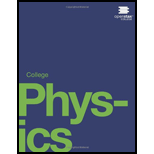# The purpose of this problem is to show in three ways that the binding energy at the election in a hydrogen atom is negligible compared with the masses of the proton and electron. (a) Calculate the mass equivalent in u of the 13.6−eV binding energy of an electron in a hydrogen atom, and compete this with the mass of the hydrogen atom obtained from Appendix A. (b) Subtract the mass at the proton given in Table 31.2 from the mass at the hydrogen atom given in Appendix A. You will find the difference is equal to the electron’s mass to three digits, implying the binding energy is small in comparison. (c) Take the ratio of the binding energy at the electron (13.6 eV) to the energy equivalent of the electron's mass (0.511 MeV). (d) Discuss how your answers confirm the stated purpose of this problem.### College Physics

1st Edition
Paul Peter Urone + 1 other
Publisher: OpenStax College
ISBN: 9781938168000### College Physics

1st Edition
Paul Peter Urone + 1 other
Publisher: OpenStax College
ISBN: 9781938168000

#### Solutions

Chapter
Section
Chapter 31, Problem 75PE
Textbook Problem

## Expert Solution

### Want to see the full answer?

Check out a sample textbook solution.See solution

### Want to see this answer and more?

Experts are waiting 24/7 to provide step-by-step solutions in as fast as 30 minutes!*

See Solution

*Response times vary by subject and question complexity. Median response time is 34 minutes and may be longer for new subjects.